• ## PLT

2017-07-19 21:57:35
PLT
Procedure Linkage Table
PLT：延迟绑定，函数第一次用到时才进行绑定（符号查找，重定位等）；
实现手法：增加一层间接跳转。
调用函数时并不直接通过GOT跳转，而是通过一个叫做PLT的项的结构来进行跳转，每个外部函数在PLT中都有一个相应的项。
ELF将GOT拆分成两个表叫做.got 和.got.plt。
.got用来保存全局变量引用的地址。
.got.plt用来保存函数引用的地址。外部函数的引用全部放到.got.plt中


展开全文• 问题当使用如下代码保存使用plt.savefig 保存生成的图片时，结果打开生成的...plt.savefig("filename.png")原因其实产生这个现象的原因很简单：在plt.show() 后调用了plt.savefig() ，在plt.show() 后实际上已经创建
更新
这里我会列出对本文的更新。
2017 年 9 月 28 日：修正几处错字，优化排版。
问题
当使用如下代码保存使用 plt.savefig 保存生成的图片时，结果打开生成的图片却是一片空白。
import matplotlib.pyplot as plt

""" 一些画图代码 """

plt.show()
plt.savefig("filename.png")

原因
其实产生这个现象的原因很简单：在 plt.show() 后调用了 plt.savefig() ，在 plt.show() 后实际上已经创建了一个新的空白的图片（坐标轴），这时候你再 plt.savefig() 就会保存这个新生成的空白图片。
解决
知道了原因，就不难知道解决办法了，解决办法有两种：

在 plt.show() 之前调用 plt.savefig()；
import matplotlib.pyplot as plt

""" 一些画图代码 """

plt.savefig("filename.png")
plt.show()


画图的时候获取当前图像（这一点非常类似于 Matlab 的句柄的概念）：
# gcf: Get Current Figure
fig = plt.gcf()
plt.show()
fig1.savefig('tessstttyyy.png', dpi=100)


END


展开全文python matplotlib
• ##plt 同时显示多幅图像 import matplotlib.pyplot as plt plt.figure() plt.subplot(1,2,1) plt.imshow(images[i]) plt.subplot(1,2,2) plt.imshow(maskes[i]) plt.show() 以下转自：...
##plt 同时显示多幅图像
import matplotlib.pyplot as plt

plt.figure()
plt.subplot(1,2,1)
plt.imshow(images[i])
plt.subplot(1,2,2)
plt.imshow(maskes[i])
plt.show()

以下转自：https://www.cnblogs.com/yinxiangnan-charles/p/5928689.html

在 python 中除了用 opencv，也可以用 matplotlib 和 PIL 这两个库操作图片。本人偏爱 matpoltlib，因为它的语法更像 matlab。

一、matplotlib

1. 显示图片

import matplotlib.pyplot as plt # plt 用于显示图片
import matplotlib.image as mpimg # mpimg 用于读取图片
import numpy as np

lena = mpimg.imread('lena.png') # 读取和代码处于同一目录下的 lena.png
# 此时 lena 就已经是一个 np.array 了，可以对它进行任意处理
lena.shape #(512, 512, 3)

plt.imshow(lena) # 显示图片
plt.axis('off') # 不显示坐标轴
plt.show()

2. 显示某个通道

# 显示图片的第一个通道
lena_1 = lena[:,:,0]
plt.imshow('lena_1')
plt.show()
# 此时会发现显示的是热量图，不是我们预想的灰度图，可以添加 cmap 参数，有如下几种添加方法：
plt.imshow('lena_1', cmap='Greys_r')
plt.show()

img = plt.imshow('lena_1')
img.set_cmap('gray') # 'hot' 是热量图
plt.show()



3. 将 RGB 转为灰度图

matplotlib 中没有合适的函数可以将 RGB 图转换为灰度图，可以根据公式自定义一个：

def rgb2gray(rgb):
return np.dot(rgb[...,:3], [0.299, 0.587, 0.114])

gray = rgb2gray(lena)
# 也可以用 plt.imshow(gray, cmap = plt.get_cmap('gray'))
plt.imshow(gray, cmap='Greys_r')
plt.axis('off')
plt.show()

4. 对图像进行放缩

这里要用到 scipy

from scipy import misc
lena_new_sz = misc.imresize(lena, 0.5) # 第二个参数如果是整数，则为百分比，如果是tuple，则为输出图像的尺寸
plt.imshow(lena_new_sz)
plt.axis('off')
plt.show()

5. 保存图像

5.1 保存 matplotlib 画出的图像

该方法适用于保存任何 matplotlib 画出的图像，相当于一个 screencapture。

plt.imshow(lena_new_sz)
plt.axis('off')
plt.savefig('lena_new_sz.png')

5.2 将 array 保存为图像

from scipy import misc
misc.imsave('lena_new_sz.png', lena_new_sz)

5.3 直接保存 array

读取之后还是可以按照前面显示数组的方法对图像进行显示，这种方法完全不会对图像质量造成损失

np.save('lena_new_sz', lena_new_sz) # 会在保存的名字后面自动加上.npy
img = np.load('lena_new_sz.npy') # 读取前面保存的数组

二、PIL

1. 显示图片

from PIL import Image
im = Image.open('lena.png')
im.show()

2. 将 PIL Image 图片转换为 numpy 数组

im_array = np.array(im)
# 也可以用 np.asarray(im) 区别是 np.array() 是深拷贝，np.asarray() 是浅拷贝

3. 保存 PIL 图片

直接调用 Image 类的 save 方法

from PIL import Image
I = Image.open('lena.png')
I.save('new_lena.png')

4. 将 numpy 数组转换为 PIL 图片

这里采用 matplotlib.image 读入图片数组，注意这里读入的数组是 float32 型的，范围是 0-1，而 PIL.Image 数据是 uinit8 型的，范围是0-255，所以要进行转换：

import matplotlib.image as mpimg
from PIL import Image
lena = mpimg.imread('lena.png') # 这里读入的数据是 float32 型的，范围是0-1
im = Image.fromarray(np.uinit8(lena*255))
im.show()

5. RGB 转换为灰度图

from PIL import Image
I = Image.open('lena.png')
I.show()
L = I.convert('L')
L.show()


展开全文• 很多时候为了可视化效果的美观，就不得不从细节上下手，这里我们就介绍一下这些细节之一的网格线。 首先导入需要用到的库，matplotlib.pyplot是必须的，Numpy是为了...plt.gcf().set_facecolor(np.ones(3)* 240 /...
很多时候为了可视化效果的美观，就不得不从细节上下手，这里我们就介绍一下这些细节之一的网格线。

首先导入需要用到的库，matplotlib.pyplot是必须的，Numpy是为了生成画布用的。

import numpy as np
import matplotlib.pyplot as plt


生成网格

plt.gcf().set_facecolor(np.ones(3)* 240 / 255)   # 生成画布的大小
plt.grid()  # 生成网格
plt.show()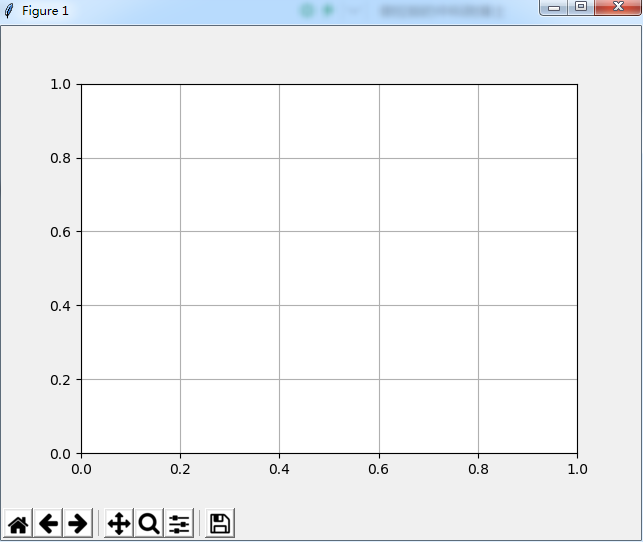参数

matplotlin.pyplot.grid(b, which, axis, color, linestyle, linewidth， **kwargs)

grid()参数有很多，这里只列举了我此次工作中用到的几个：

b : 布尔值。就是是否显示网格线的意思。官网说如果b设置为None， 且kwargs长度为0，则切换网格状态。但是没弄明白什            么意思。如果b设置为None，但是又给了其它参数，则默认None值失效。

which : 取值为'major', 'minor'， 'both'。 默认为'major'。看别人说是显示的，我的是Windows7下，用Sublime跑的，minor                 只是一个白画板，没有网格，major和both也没看出什么效果，不知道为什么。

axis : 取值为‘both’， ‘x’，‘y’。就是想绘制哪个方向的网格线。不过我在输入参数的时候发现如果输入x或y的时候，             输入的是哪条轴，则会隐藏哪条轴

color : 这就不用多说了，就是设置网格线的颜色。或者直接用c来代替color也可以。

linestyle :也可以用ls来代替linestyle， 设置网格线的风格，是连续实线，虚线或者其它不同的线条。 | '-' | '--'                        | '-.' | ':' | 'None' | ' ' | '']

linewidth : 设置网格线的宽度

设置axis='y'

plt.grid(axis="y")
plt.show()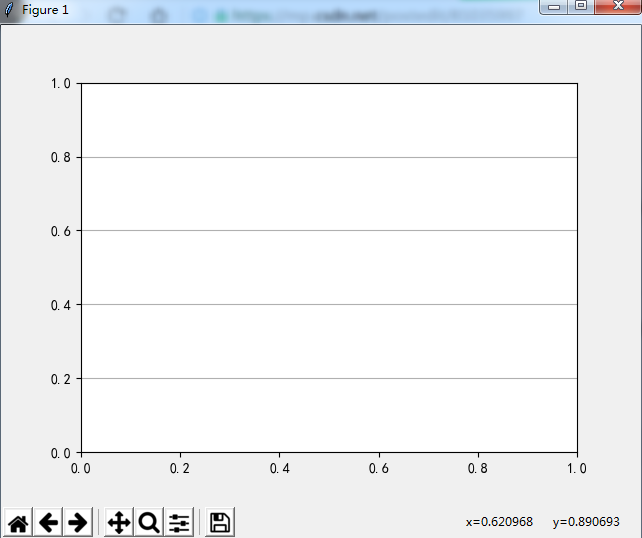设置axis='x'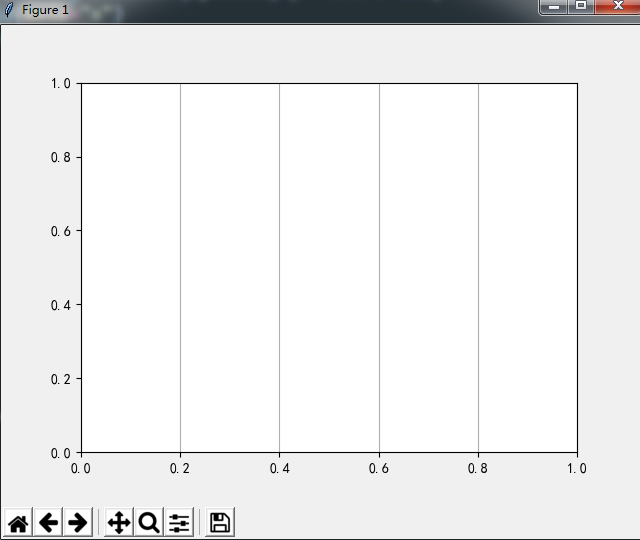设置color='r'

plt.grid(c='r')
plt.show()

红色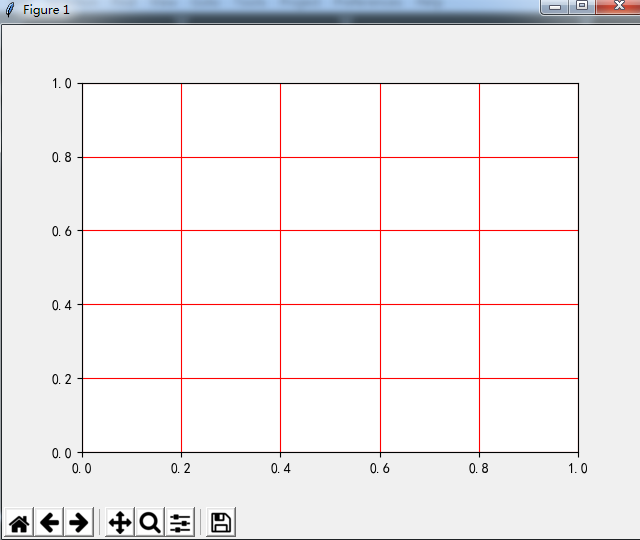plt.grid(c='g')
plt.show()

绿色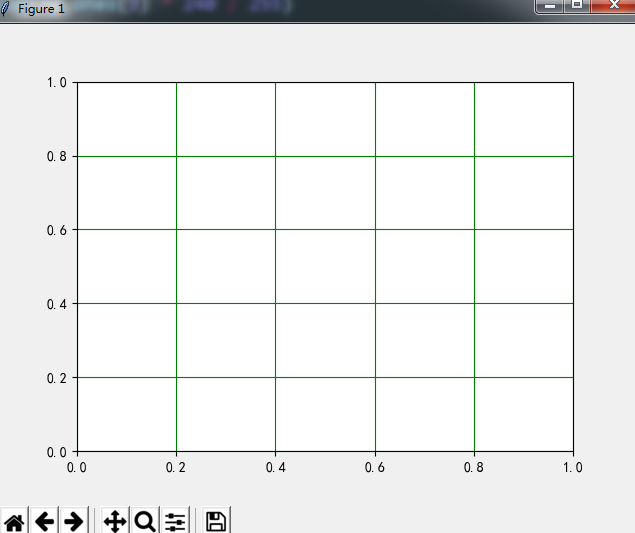设置linestyle

plt.grid(linestyle='-.')
plt.show()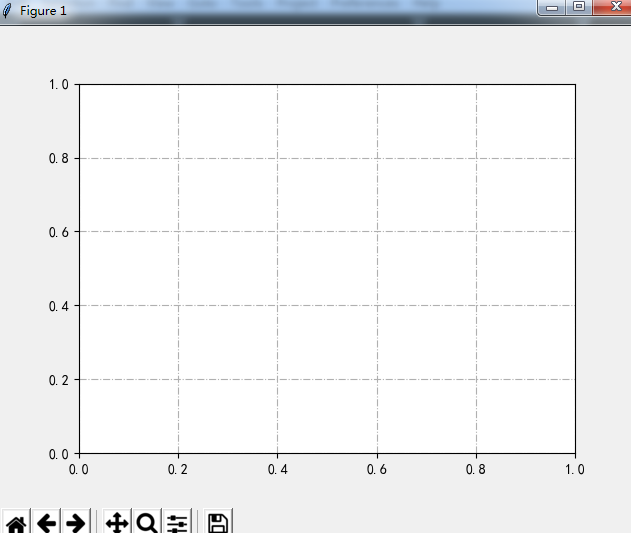plt.grid(ls='--')
plt.show()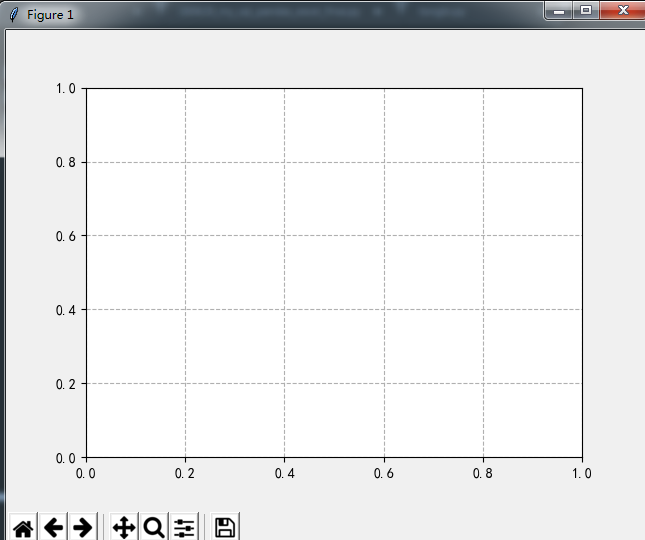因为b和which没有显示效果。所以这里就不上图了。

--------------------------更******新--------------------------

今天又试了下，当which='major'的时候，是可以显示网格线的。同时感谢机器不学习o_o的指导，在which="minor"时，需要设置次刻度线。

plt.grid(axis='y', which='major')
plt.show()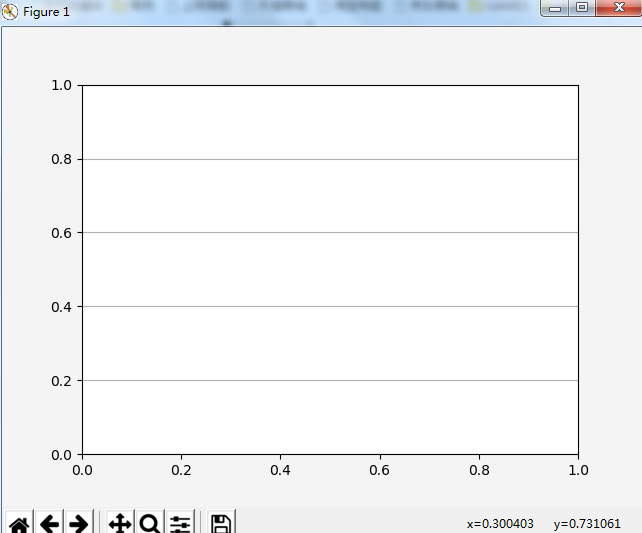plt.grid(axis="x", which="major")
plt.show()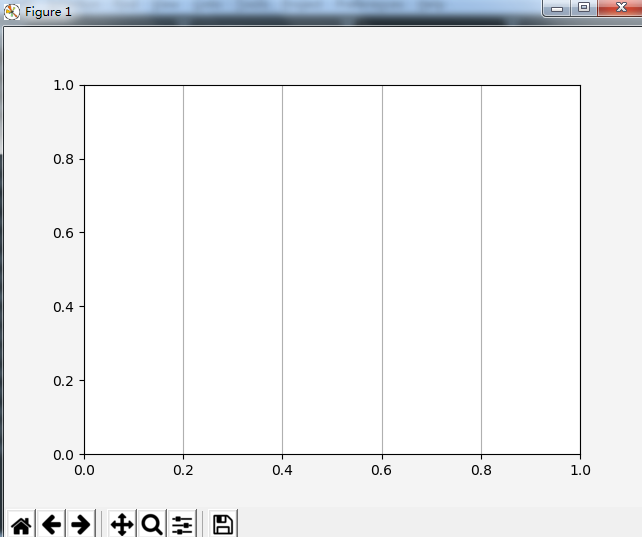which='minor'

ax = plt.gca()
ax.set_xlim(0, 10)
miloc = plt.MultipleLocator(1)
ax.xaxis.set_minor_locator(miloc)
ax.grid(axis='x', which='minor')
plt.show()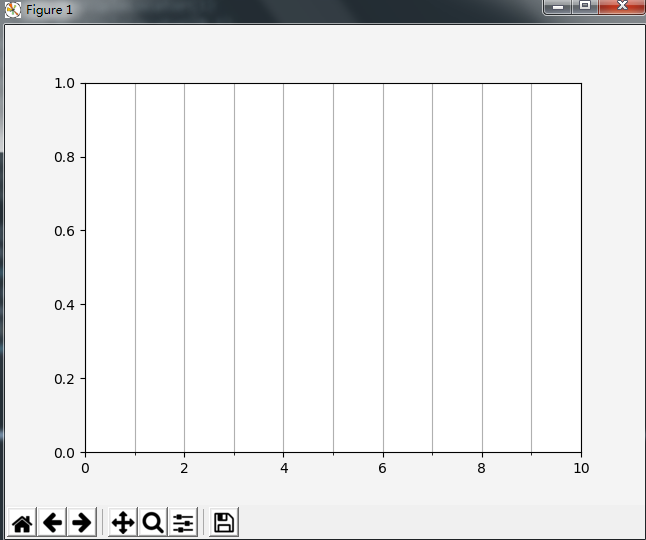展开全文• plt contour 与 plt contourf
• ## plt.plot()的使用方法以及参数介绍

万次阅读 多人点赞 2019-05-21 09:07:48
偶然的一次操作，对plt.plot()中，仅仅传入了一个列表，然后逻辑就和预想的出现了偏差。 plt.plot() plt.plot() 参数介绍： x, y : array-like or scalar The horizontal / vertical coordinates of the data ...matplotlib python pyplot
• plt.plotfig = plt.figure(figsize=(16,16),dpi=300) # 初始化一张画布，可以设置figsize和dpiplt.plot() #直接在一张...设计模式 多线程 redis nginx js
• ## plt.imshow()与plt.show()区别

万次阅读 多人点赞 2019-01-04 17:17:24
plt.imshow()函数负责对图像进行处理，并显示其格式，但是不能显示。 其后跟着plt.show()才能显示出来。
• for path1, path2 in same: plt.figure() im1 = plt.imread(path1) im2 = plt.imread(path2) plt.subplot(1,2,1) plt.imshow(im1) plt.subplot(1,2,2) plt.imshow(im2) plt.show() ...
• 一、fig, ax = plt.subplots()的作用？ 二、参数的含义？ 三、怎么在一个图上排列多个子图？ 四、怎么把多个子图一起合并到一个图上？ 《Python for Data Analysis》 2nd Edition 一、fig, ax = plt.subplots()...python fig 格式
• ## plt.show()和plt.imshow()的区别

万次阅读 多人点赞 2019-04-15 10:15:03
问题：plt.imshow()无法显示图像 解决方法:添加：plt.show()，即 plt.imshow(image) #image表示待处理的图像 plt.show() 原理：plt.imshow()函数负责对图像进行处理，并显示其格式，而plt.show()则是将plt....python
• ## PYthon——plt.scatter各参数详解

万次阅读 多人点赞 2017-03-29 21:22:47
matplotlib.pyplot as plt  #产生测试数据   x = np.arange(1,10)  y = x  fig = plt.figure()  ax1 = fig.add_subplot(111)  #设置标题   ax1.set_title( 'Scatter Plot'...python 机器学习 编程语言 散点图
• ## plt.rcParams参数详解

万次阅读 多人点赞 2020-03-10 17:11:44
• ## plt.subplot()使用方法以及参数介绍

万次阅读 多人点赞 2019-05-25 08:56:12
plt.subplot() plt.subplot(nrows, ncols, index, **kwargs) 第一个参数：*args （官网文档描述） Either a 3-digit integer or three separate integers describing the position of the subplot. If the three ...matplotlib python 数据分析
• 以下内容中所有plt均为matplotlib.pyplot的简称。1、标题可以用函数plt.title()来设置图形标题。以下代码中绘制了折线图，并给其设置了标题。import matplotlib.pyplot as pltimport numpy as npplt.rcParams['font....
• plt.imshow()函数负责对图像进行处理，并显示其格式。 plt.show()则是将plt.imshow()处理后的函数显示出来。 所以会出现plt.imshow不显示图像的问题 解决方法： 在plt.imshow()后面加上一个plt.show() ...python pycharm
• ____tz_zs 关于这些方法的使用见 matplotlib 模块的简单使用 ，本文是关于这些方法在源码中的调用过程，用以...plt.plot、plt.scatter、plt.hist、plt.bar 等方法均调用 gcf() 获取 figure 对象，然后调用 figu...pyplot
• plt.figure(figsize=(6,8)) 表示figure 的大小为宽、长（单位为inch） figsize : (float, float), optional, default: None width, height in inches. If not provided, defaults to rcParams[“figure.figsize”] =...
• 1.设置x y值 x = np.arange(0,100,0.01) y = 100/((100-x)+(x/...plt.xlabel('Percent vectorization') plt.ylabel('Net Speedup') 3.x y 轴范围 plt.xlim(0,100) plt.ylim(0,10) 4.图像名称 plt.title('Plot of th...
• ## python plt 绘图

千次阅读 2018-10-12 09:55:25
plt import matplotlib.pyplot as plt fig = plt.figure() 创建一个画布 plt.show() 显示图像一般用在最后 plt.savefig(‘confusion_matrix.png’, format=‘png’) plt.title(title) plt.co...
• ## plt label生效

千次阅读 2019-05-14 18:03:31
fig = plt.figure() fig.set_size_inches(12, 8) # ts1.plot(label="after") plt.plot(ts1,label="after") plt.legend() plt.show() 代码段如上，如果按照注释上的写，label就不会生效，而且最后还要加上“plg....
• contour：轮廓，等高线。...plt.contourf 与 plt.contour 区别：f：filled，也即对等高线间的填充区域进行填充（使用不同的颜色）； contourf：将不会再绘制等高线（显然不同的颜色分界就表示等高线本身）， 增加
• plt.subplots(nrows, ncols) 子图需要一个一个的指定 plt.subplot(nrows, ncols, i) 子图可以使用for循环 一. plt.subplots() fig, axes = plt.subplots(nrows = 3, ncols = 2, figsize = (12, 12)) sns.boxplot(.....python
• ## python plt 色卡

千次阅读 2019-08-30 09:56:28
plt 绘图时通常需要各种颜色，还需要去介绍文档找，很麻烦，这里把plt的色卡单独列出来，方便查看使用。
• 二者都可以显示图像，plt.show显示的是plt绘制的图像，而plt.imshow显示的是调用出的二进制矩阵的图像。 例如python
•matplotlib
• matplotlib1、plt.plot(x,y)plt.plot(x,y,format_string,**kwargs) x轴数据，y轴数据，format_string控制曲线的格式字串 format_string 由颜色字符，风格字符，和标记字符import matplotlib.pyplot as pltplt.plot......